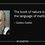Let's define a function f(x,y,z) ,where (x,y,z) are points in 3-d space.Let's take value of our function at some point in space (x,y,z) . Now on moving from this point to SOME point in near by space, gives us maximum rate of change to our function .WHICH point????????
Answer to this question is given by Gradient of that function. Mathematically,Gradient of a function is a vector that represents both the magnitude and direction of maximum space rate of increase of that function.
gradient of function ∇ f= ∂ f/ ∂ x Ax + ∂ f/∂ y Ay +∂ f/∂ z Az.

• Now,rate of change of our function "f" in 3-D space along any direction can be found by multiplying magnitude of gradient vector with cosine of angle b/w given direction and gradient vector.!!!!.This is called DIRECTIONAL DERIVATIVE .

-Now,consider a region in 3d space where magnitude of our function remains same.this region or surface is called equipotential surface.Here rate of change of function is zero. Applying above result , |∇f|COSΘ=0 . So,Gradient of a function is perpendicular to equipotential surface !!!!.Note by Thushar Mn
6 years, 5 months ago

This discussion board is a place to discuss our Daily Challenges and the math and science related to those challenges. Explanations are more than just a solution — they should explain the steps and thinking strategies that you used to obtain the solution. Comments should further the discussion of math and science.

When posting on Brilliant:

• Use the emojis to react to an explanation, whether you're congratulating a job well done , or just really confused .
• Ask specific questions about the challenge or the steps in somebody's explanation. Well-posed questions can add a lot to the discussion, but posting "I don't understand!" doesn't help anyone.
• Try to contribute something new to the discussion, whether it is an extension, generalization or other idea related to the challenge.

MarkdownAppears as
*italics* or _italics_ italics
**bold** or __bold__ bold
- bulleted- list
• bulleted
• list
1. numbered2. list
1. numbered
2. list
Note: you must add a full line of space before and after lists for them to show up correctly
paragraph 1paragraph 2

paragraph 1

paragraph 2

[example link](https://brilliant.org)example link
> This is a quote
This is a quote
    # I indented these lines
# 4 spaces, and now they show
# up as a code block.

print "hello world"
# I indented these lines
# 4 spaces, and now they show
# up as a code block.

print "hello world"
MathAppears as
Remember to wrap math in $$ ... $$ or $ ... $ to ensure proper formatting.
2 \times 3 $2 \times 3$
2^{34} $2^{34}$
a_{i-1} $a_{i-1}$
\frac{2}{3} $\frac{2}{3}$
\sqrt{2} $\sqrt{2}$
\sum_{i=1}^3 $\sum_{i=1}^3$
\sin \theta $\sin \theta$
\boxed{123} $\boxed{123}$